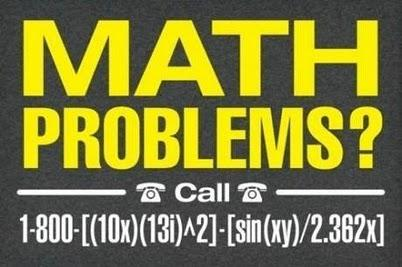# MATH PROBLEMS?

Maths in the City posted this on twitter:In order to make a number we can call, we need both of $n=(10x)(13i^2)$ and $m=\frac{\sin(xy)}{2.362x}$ to be integers.

Multiply out the first one:

$(10x)(13i)^2 = -1690x$

So $$x$$ needs to be of the form

$-\frac{n}{1690},$

Now, to look at the second part!

Substituting in the expression we had for $$x$$, we get

$m = \frac{\sin \left( \left(\frac{-n}{1690} \right) y \right)}{2.362 \left(\frac{-n}{1690} \right)}.$

Let’s rearrange that to find $$y$$ in terms of $$m$$ and $$n$$:

\begin{align} \frac{-2.362mn}{1690}&= \sin \left( \frac{-ny}{1690} \right) \\ \sin^{-1}\left(\frac{-2.362mn}{1690} \right) &= -\frac{ny}{1690}\end{align}

At this point, note that $$\sin(-z) = -\sin(z).$$

\begin{align} \frac{ny}{1690} &= \sin^{-1}\left( \frac{2.362mn}{1690}\right) \\ \\ \\ y &= \left( \frac{1690}{n} \right) \sin^{-1}\left( \frac{2.362mn}{1690}\right)\end{align}

Which is great!

Since $$\sin^{-1}$$ is defined only on values in the range $$[-1,1]$$, and we want both $$m$$ and $$n$$ to be positive, we need

$0 \leq \frac{2.362mn}{1690} \leq 1$

i.e.

$mn \leq \frac{1690}{2.362},$

or,

$mn \leq 715.495343.$

So if you have “math problems”, call any number 1-800-n-m such that $$mn \leq 715$$.

$i$ is the square root of $-1$, i.e. $i^2 = -1$, so it’s the minus sign.